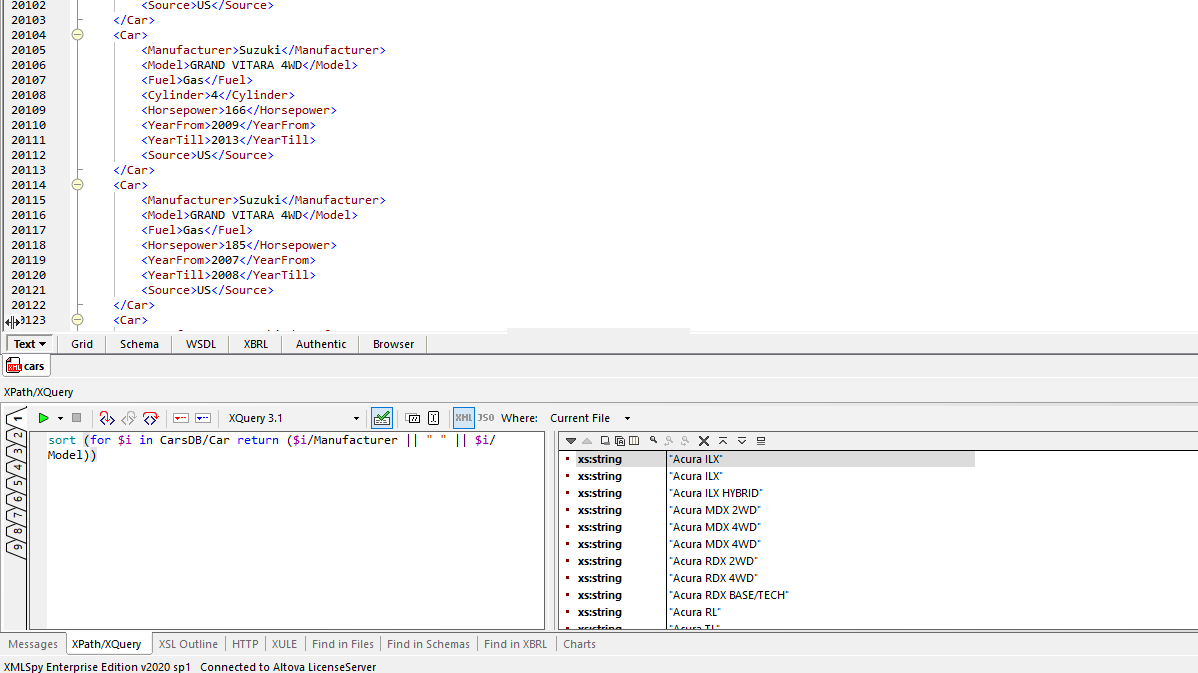## XPath/XQuery sort function

### Summary

Sorts a supplied sequence, based on the value of a sort key supplied as a function.

### Signatures

`fn:sort`(
`\$input` as `item()*`
) as `item()*`
`fn:sort`(
`\$input` as `item()*`,
`\$collation` as `xs:string?`
) as `item()*`
`fn:sort`(
`\$input` as `item()*`,
`\$collation` as `xs:string?`,
`\$key` as `function(item()) as xs:anyAtomicType*`
) as `item()*`

### Properties

The one-argument form of this function is deterministic, context-dependent, and focus-independent. It depends on collations.
The two-argument form of this function is deterministic, context-dependent, and focus-independent. It depends on collations.
The three-argument form of this function is deterministic, context-dependent, focus-independent, and higher-order. It depends on collations.

### Rules

Calling the single-argument version of the function is equivalent to calling the two-argument form with `default-collation()` as the second argument: that is, it sorts a sequence of items according to the typed value of the items, using the default collation to compare strings.

Calling the two-argument version of the function is equivalent to calling the three-argument form with `fn:data#1` as the third argument: that is, it sorts a sequence of items according to the typed value of the items, using a specified collation to compare strings.

In the case of both `fn:sort#2` and `fn:sort#3`, supplying an empty sequence as the second argument is equivalent to supplying `fn:default-collation()`. For more information on collations see .

The result of the function is obtained as follows:

• For each item in the sequence `\$input`, the function supplied as `\$key` is evaluated with that item as its argument. The resulting values are the sort keys of the items in the input sequence.

• The result sequence contains the same items as the input sequence `\$input`, but generally in a different order.

• Let \$C be the selected collation, or the default collation where applicable.

• The order of items in the result is such that, given two items `\$A` and `\$B`:

If `(fn:deep-equal(\$key(\$A), \$key(\$B), \$C)`, then the relative order of `\$A` and `\$B` in the output is the same as their relative order in the input (that is, the sort is stable)

Otherwise, if `(deep-less-than(\$key(\$A), \$key(\$B), \$C)`, then `\$A` precedes `\$B` in the output. The function `deep-less-than` is defined as the boolean result of the expression:

```if (fn:empty(\$A)) then fn:exists(\$B) else if (fn:deep-equal(\$A, \$B, \$C)) then deep-less-than(fn:tail(\$A), fn:tail(\$B), \$C) else if (\$A ne \$A (:that is, \$A is NaN:)) then fn:true() else if (is-string(\$A) and is-string(\$B) then fn:compare(\$A, \$B, \$C) lt 0 else \$A lt \$B```

where the function `is-string(\$X)` returns true if and only if `\$X` is an instance of `xs:string`, `xs:anyURI`, or `xs:untypedAtomic`.

This ordering of sequences is referred to by mathematicians as "lexicographic ordering".

### Examples

The expression `fn:sort((1, 4, 6, 5, 3))` returns `(1, 3, 4, 5, 6)`.

The expression `fn:sort((1, -2, 5, 10, -10, 10, 8), (), fn:abs#1)` returns `(1, -2, 5, 8, 10, -10, 10)`.

To sort a set of strings `\$in` using Swedish collation:

let \$SWEDISH := "http://www.w3.org/2013/collation/UCA?lang=se" return fn:sort(\$in, \$SWEDISH)

To sort a sequence of employees by last name as the major sort key and first name as the minor sort key, using the default collation:

fn:sort(\$employees, (), function(\$emp) {\$emp/name ! (last, first)})### Error Conditions

If the set of computed sort keys contains values that are not comparable using the `lt` operator then the sort operation will fail with a type error ().

### Notes

XSLT and XQuery both provide native sorting capability, but previous releases of XPath provided no sorting functionality for use in standalone environments.

In addition there are cases where this function may be more flexible than the built-in sorting capability for XQuery or XSLT, for example when the sort key or collation is chosen dynamically, or when the sort key is a sequence of items rather than a single item.

The results are compatible with the results of XSLT sorting (using `xsl:sort`) in the case where the sort key evaluates to a sequence of length zero or one, given the options `stable="yes"` and `order="ascending"`.

The results are compatible with the results of XQuery sorting (using the `order by` clause) in the case where the sort key evaluates to a sequence of length zero or one, given the options `stable`, `ascending`, and `empty least`.